# Berezin transform

(diff) ← Older revision | Latest revision (diff) | Newer revision → (diff)

Berezin transformation

The Berezin transform associates smooth functions with operators on Hilbert spaces of analytic functions. The usual setting involves an open setand a Hilbert space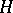of analytic functions on(cf. also Analytic function). It is assumed that, for each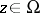, the point evaluation atis a continuous linear functional on. Thus, for each, there exists a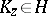such that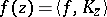for every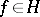. Becausereproduces the value of functions inat, it is called the reproducing kernel. The normalized reproducing kernel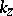is defined by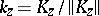.

Fora bounded operator on, the Berezin transform of, denoted by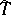, is the complex-valued function ondefined by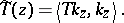For each bounded operatoron, the Berezin transformis a bounded real-analytic function on. Properties of the operatorare often reflected in properties of the Berezin transform.

The Berezin transform is named in honour of F. Berezin, who introduced this concept in [a4].

The Berezin transform has been useful in several contexts, ranging from the Hardy space (see, for example, [a8]) to the Bargmann–Segal space (see, for example, [a5]), with major connections to the Bloch space and functions of bounded mean oscillation (see, for example, [a9]). However, the Berezin transform has been most successful as a tool to study operators on the Bergman space. For concreteness and simplicity, attention below is restricted to the latter setting.

The Bergman space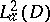(cf. also Bergman spaces) consists of the analytic functionson the unit disc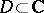such that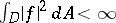(here,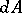denotes area measure, normalized so that the area ofequals). The normalized reproducing kernel is then given by the formula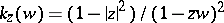.

For, the Toeplitz operator with symbolis the operatorondefined by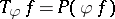, whereis the orthogonal projection ofonto(cf. also Toeplitz operator). The Berezin transform of the function, denoted by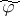, is defined to be the Berezin transform of the Toeplitz operator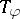. This definition easily leads to the formula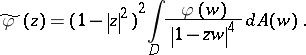Ifis a bounded harmonic function on, then the mean-value property can be used to show that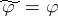. The converse was proved by M. Engliš [a6]: if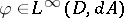and, thenis harmonic on. P. Ahern, M. Flores and W. Rudin [a1] extended this result to functions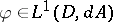(the formula above formakes sense in this case) and showed that the higher-dimensional analogue is valid up to dimension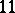but fails in dimensions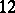and beyond.

The normalized reproducing kerneltends weakly to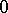as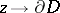. This implies that ifis a compact operator on the Bergman space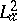, then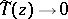as. Unfortunately, the converse fails. For example, ifis the operator ondefined by, then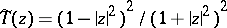. Thus, in this caseas, butis not compact (in fact, this operatoris unitary, cf. also Unitary operator).

However, the situation is much nicer for Toeplitz operators, and even, more generally, for finite sums of finite products of Toeplitz operators. S. Axler and D. Zheng [a2] proved that such an operator is compact if and only if its Berezin transform tends toat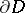.

The Berezin transform also makes an appearance in the decomposition of the Toeplitz algebragenerated by the Toeplitz operators with analytic symbol. Specifically, G. McDonald and C. Sundberg [a7] proved that if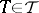, thencan be written in the form, where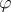is in the closed algebra generated by the bounded harmonic functions on the unit disc andis in the commutator ideal of. The choice ofis not unique, but takingto be the Berezin transform ofalways works (see [a3]).

How to Cite This Entry:
Berezin transform. Encyclopedia of Mathematics. URL: http://encyclopediaofmath.org/index.php?title=Berezin_transform&oldid=12000
This article was adapted from an original article by Sheldon Axler (originator), which appeared in Encyclopedia of Mathematics - ISBN 1402006098. See original article